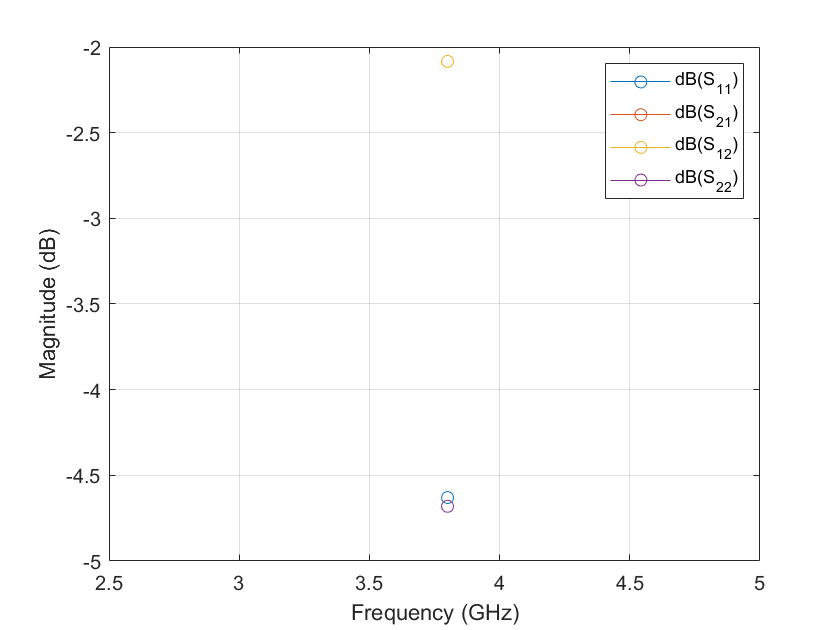# interdigitalCapacitor

Create basic interdigital capacitor

Since R2021b

## Description

Use the `interdigitalCapacitor` object to create an interdigital planar capacitor (IDC). IDCs are used in high frequency applications such as:

• Wireless data communications with RFID

• Humidity and solution concentration measurements

• Lab-on-chip devices (LOCs)

Note

This PCB object supports behavioral modeling. For more information, see Behavioral Models. To analyze the behavioral model for a interdigital capacitor, set the `Behavioral` property in the `sparameters` function to `true or 1`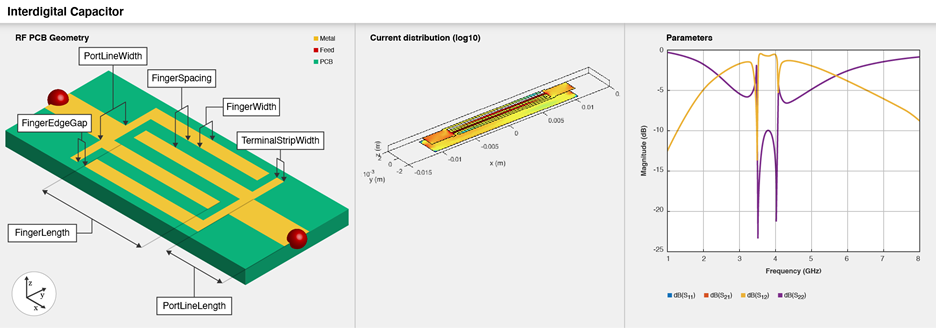A two-port series IDC with microstrip form feeder lines supports single and multiple dielectrics. It is a coplanar structure consisting of multiple comb electrodes or intersecting fingers with spaces between the fingers. An IDC can have identical port line lengths and widths on either sides.

## Creation

### Syntax

``capacitor = interdigitalCapacitor``
``capacitor = interdigitalCapacitor(Name=Value)``

### Description

example

````capacitor = interdigitalCapacitor` creates a basic interdigital capacitor with default property values for an operating bandwidth of 3.6-4 GHz.```

example

````capacitor = interdigitalCapacitor(Name=Value)` sets Properties using one or more name-value arguments. For example, `interdigitalCapacitor(NumFingers=10)` creates an interdigital capacitor with 10 fingers. Properties not specified retain their default values.```

## Properties

expand all

Number of fingers on the capacitor, specified as a positive scalar.

Example: `capacitor = interdigitalCapacitor(NumFingers=10)`

Data Types: `double`

Length of the overlapping fingers in meters, specified as a positive scalar.

Example: `capacitor = interdigitalCapacitor(FingerLength=0.0217)`

Data Types: `double`

Width of the overlapping fingers in meters, specified as a positive scalar.

Example: ```capacitor = interdigitalCapacitor(FingerWidth=4.8000e-04)```

Data Types: `double`

Distance between the fingers in meters, specified as a positive scalar.

Example: ```capacitor = interdigitalCapacitor(FingerSpacing=2.9000e-04)```

Data Types: `double`

Gap between the edges of the fingers in meters, specified as a positive scalar.

Example: ```capacitor = interdigitalCapacitor(FingerEdgeGap=2.05000e-04)```

Data Types: `double`

Width of the terminals in meters, specified as a positive scalar.

Example: ```capacitor = interdigitalCapacitor(TerminalStripWidth=4.9000e-04)```

Data Types: `double`

Width of the ports in meters, specified as a positive scalar.

Example: ```capacitor = interdigitalCapacitor(PortLineWidth=0.0020)```

Data Types: `double`

Length of the ports in meters, specified as a positive scalar.

Example: ```capacitor = interdigitalCapacitor(PortLineLength=0.0040)```

Data Types: `double`

Height from the capacitor to the ground plane in meters, specified as a positive scalar.

Example: `capacitor = interdigitalCapacitor(Height=6.9000e-04)`

Data Types: `double`

Width of the ground plane in meters, specified as a positive scalar.

Example: ```capacitor = interdigitalCapacitor(GroundPlaneWidth=0.0040)```

Example: double

Type of dielectric material used as a substrate, specified as a `dielectric` object. The dielectric material in a `interdigitalCapacitor` with default properties have the following properties:

• `Name``{'Roger'}`

• `EpsilonR``3.2`

• `LossTangent``0.0002`

• `Thickness``0.000787`

Example: `d = dielectric("FR4");` `capacitor = interdigitalCapacitor(Substrate=d)`

Data Types: `string` | `char`

Type of metal used in the conducting layers, specified as a `metal` object. he type of metal in a `couplerBranchlineWideband` object with default properties is Copper.

Example: `m = metal("PEC");` `capacitor =interdigitalCapacitor(Conductor=m)`

Data Types: `string` | `char`

## Object Functions

 `capacitance` Calculate capacitance `charge` Calculate and plot charge distribution `current` Calculate and plot current distribution `feedCurrent` Calculate current at feed port `getZ0` Calculate characteristic impedance of transmission line `layout` Plot all metal layers and board shape `mesh` Change and view mesh properties of metal or dielectric in PCB component `shapes` Extract all metal layer shapes of PCB component `show` Display PCB component structure or PCB shape `sparameters` Calculate S-parameters for RF PCB objects

## Examples

collapse all

Create and view a default interdigital capacitor.

`idcapacitor = interdigitalCapacitor`
```idcapacitor = interdigitalCapacitor with properties: NumFingers: 4 FingerLength: 0.0137 FingerWidth: 3.1600e-04 FingerSpacing: 3.0000e-04 FingerEdgeGap: 3.4100e-04 TerminalStripWidth: 5.0000e-04 PortLineWidth: 0.0019 PortLineLength: 0.0030 Height: 7.8700e-04 GroundPlaneWidth: 0.0030 Substrate: [1x1 dielectric] Conductor: [1x1 metal] ```
`show(idcapacitor)`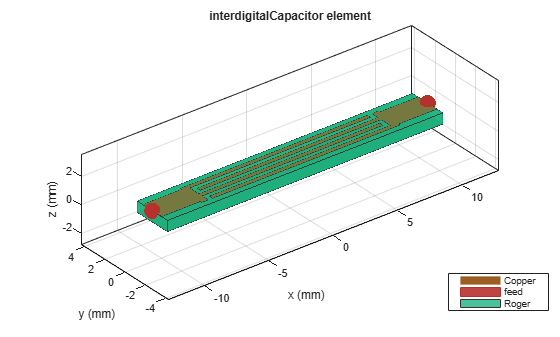Create and view a multilayer interdigital capacitor with two different dielectrics.

```idcapacitor = interdigitalCapacitor; sub = dielectric("FR4","Teflon"); sub.Thickness =[0.00003 0.00003]; idcapacitor.Substrate = sub; idcapacitor.Height = 0.00003; show(idcapacitor);```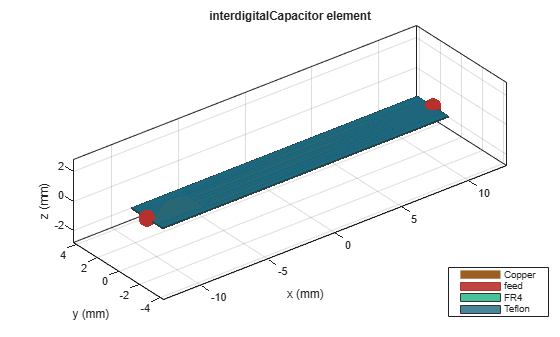Create an interdigital capacitor using gold as the conductor.

```capacitor = interdigitalCapacitor; capacitor.Conductor = metal("Gold"); show(capacitor)```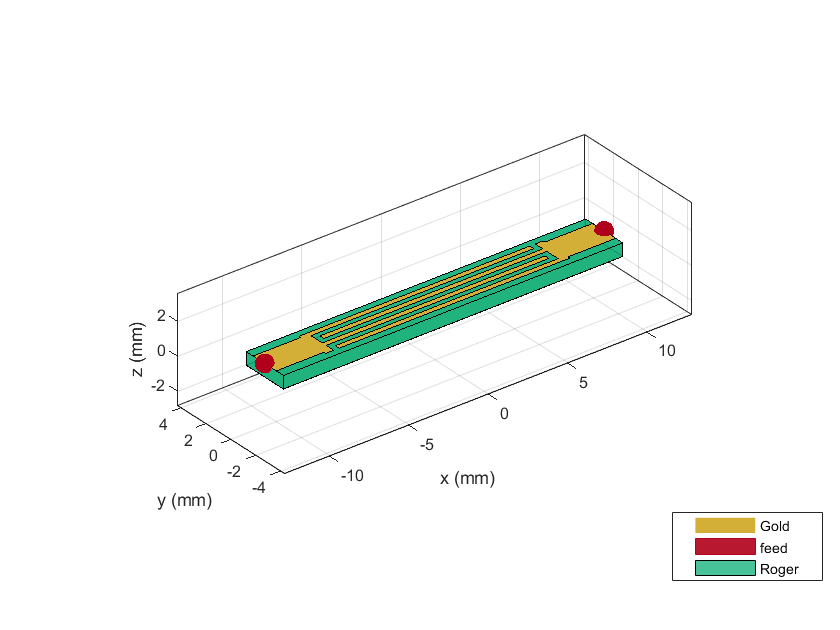Compute and plot the behavioral S-parameters of the capacitor at 3.8 GHz.

```spar = sparameters(capacitor,3.8e9,Behavioral=true); rfplot(spar)```